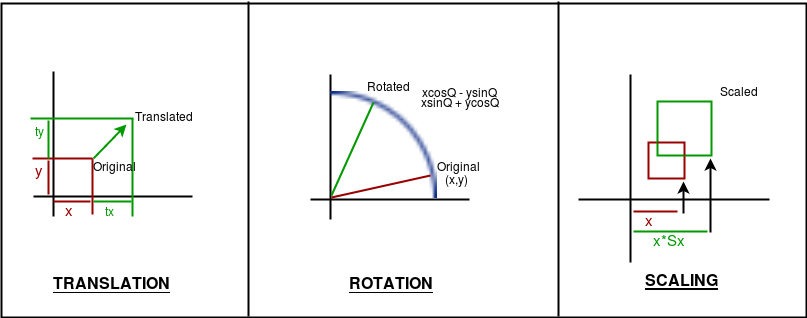Related Articles
Basic Transformations in OPENGL
• Last Updated : 08 Feb, 2018

Transformations play a very important role in manipulating objects on screen. It should be noted that here the algorithms will be implemented in code and the built-in functions will not be used to give a good understanding of how the algorithms work.
Also note that all transformations are implemented in 2D.
There are three basic kinds of Transformations in Computer Graphics:
1. Translation
2. Rotation
3. ScalingAlgorithms

1. Translation : Translation refers to moving an object to a different position on screen.
```Formula:  X = x + tx
Y = y + ty
where tx and ty are translation coordinates

The OpenGL function is glTranslatef( tx, ty, tz );```
2. Rotation : Rotation refers to rotating a point.
```Formula:  X = xcosA - ysinA
Y = xsinA + ycosA,
A is the angle of rotation.
The above formula will rotate the point around the origin.
To rotate around a different point, the formula:
X = cx + (x-cx)*cosA - (y-cy)*sinA,
Y = cx + (x-cx)*sinA + (y-cy)*cosA,
cx, cy is centre coordinates,
A is the angle of rotation.

The OpenGL function is glRotatef (A, x, y, z). ```
3. Scaling : Scaling refers to zooming in and out an object in different scales across axes.
```Formula: X = x*sx
Y = y*sy,    sx, sy being scaling factors.

The OpenGL function is glScalef(float x, float y, float z)
```

Note: If combined transformations is to be applied, follow the order: translate, rotate, scale

Implementation

 `// C code to implement basic``// transformations in OPENGL``#include ``#include ``#include ``#include `` ` `// window size``#define maxWD 640``#define maxHT 480`` ` `// rotation speed``#define thetaSpeed 0.05`` ` `// this creates delay between two actions``void` `delay(unsigned ``int` `mseconds)``{``    ``clock_t` `goal = mseconds + ``clock``();``    ``while` `(goal > ``clock``())``        ``;``}`` ` `// this is a basic init for the glut window``void` `myInit(``void``)``{``    ``glClearColor(1.0, 1.0, 1.0, 0.0);``    ``glMatrixMode(GL_PROJECTION);``    ``glLoadIdentity();``    ``gluOrtho2D(0.0, maxWD, 0.0, maxHT);``    ``glClear(GL_COLOR_BUFFER_BIT);``    ``glFlush();``}`` ` `// this function just draws a point``void` `drawPoint(``int` `x, ``int` `y)``{``    ``glPointSize(7.0);``    ``glColor3f(0.0f, 0.0f, 1.0f);``    ``glBegin(GL_POINTS);``    ``glVertex2i(x, y);``    ``glEnd();``}`` ` `void` `rotateAroundPt(``int` `px, ``int` `py, ``int` `cx, ``int` `cy)``{``    ``float` `theta = 0.0;``    ``while` `(1) {``        ``glClear(GL_COLOR_BUFFER_BIT);``        ``int` `xf, yf;`` ` `        ``// update theta anticlockwise rotation``        ``theta = theta + thetaSpeed;`` ` `        ``// check overflow``        ``if` `(theta >= (2.0 * 3.14159))``            ``theta = theta - (2.0 * 3.14159);`` ` `        ``// actual calculations..``        ``xf = cx + (``int``)((``float``)(px - cx) * ``cos``(theta))``             ``- ((``float``)(py - cy) * ``sin``(theta));``        ``yf = cy + (``int``)((``float``)(px - cx) * ``sin``(theta))``             ``+ ((``float``)(py - cy) * ``cos``(theta));`` ` `        ``// drawing the centre point``        ``drawPoint(cx, cy);`` ` `        ``// drawing the rotating point``        ``drawPoint(xf, yf);``        ``glFlush();``        ``// creating a delay``        ``// so that the point can be noticed``        ``delay(10);``    ``}``}`` ` `// this function will translate the point``void` `translatePoint(``int` `px, ``int` `py, ``int` `tx, ``int` `ty)``{``    ``int` `fx = px, fy = py;``    ``while` `(1) {``        ``glClear(GL_COLOR_BUFFER_BIT);`` ` `        ``// update``        ``px = px + tx;``        ``py = py + ty;`` ` `        ``// check overflow to keep point in screen``        ``if` `(px > maxWD || px < 0 || py > maxHT || py < 0) {``            ``px = fx;``            ``py = fy;``        ``}`` ` `        ``drawPoint(px, py); ``// drawing the point`` ` `        ``glFlush();``        ``// creating a delay``        ``// so that the point can be noticed``        ``delay(10);``    ``}``}`` ` `// this function draws``void` `scalePoint(``int` `px, ``int` `py, ``int` `sx, ``int` `sy)``{``    ``int` `fx, fy;``    ``while` `(1) {``        ``glClear(GL_COLOR_BUFFER_BIT);`` ` `        ``// update``        ``fx = px * sx;``        ``fy = py * sy;`` ` `        ``drawPoint(fx, fy); ``// drawing the point`` ` `        ``glFlush();``        ``// creating a delay``        ``// so that the point can be noticed``        ``delay(500);`` ` `        ``glClear(GL_COLOR_BUFFER_BIT);`` ` `        ``// update``        ``fx = px;``        ``fy = py;`` ` `        ``// drawing the point``        ``drawPoint(fx, fy);``        ``glFlush();``        ``// creating a delay``        ``// so that the point can be noticed``        ``delay(500);``    ``}``}`` ` `// Actual display function``void` `myDisplay(``void``)``{``    ``int` `opt;``    ``printf``(``"\nEnter\n\t<1> for translation"``           ``"\n\t<2> for rotation"``           ``"\n\t<3> for scaling\n\t:"``);``    ``scanf``(``"%d"``, &opt);``    ``printf``(``"\nGo to the window..."``);``    ``switch` `(opt) {``    ``case` `1:``        ``translatePoint(100, 200, 1, 5);``        ``break``;``    ``case` `2:``        ``rotateAroundPt(200, 200, maxWD / 2, maxHT / 2);``        ``// point will circle around``        ``// the centre of the window``        ``break``;``    ``case` `3:``        ``scalePoint(10, 20, 2, 3);``        ``break``;``    ``}``}`` ` `void` `main(``int` `argc, ``char``** argv)``{``    ``glutInit(&argc, argv);``    ``glutInitDisplayMode(GLUT_SINGLE | GLUT_RGB);``    ``glutInitWindowSize(maxWD, maxHT);``    ``glutInitWindowPosition(100, 150);``    ``glutCreateWindow(``"Transforming point"``);``    ``glutDisplayFunc(myDisplay);``    ``myInit();``    ``glutMainLoop();``}`

This article is contributed by Suprotik Dey. If you like GeeksforGeeks and would like to contribute, you can also write an article using contribute.geeksforgeeks.org or mail your article to contribute@geeksforgeeks.org. See your article appearing on the GeeksforGeeks main page and help other Geeks.